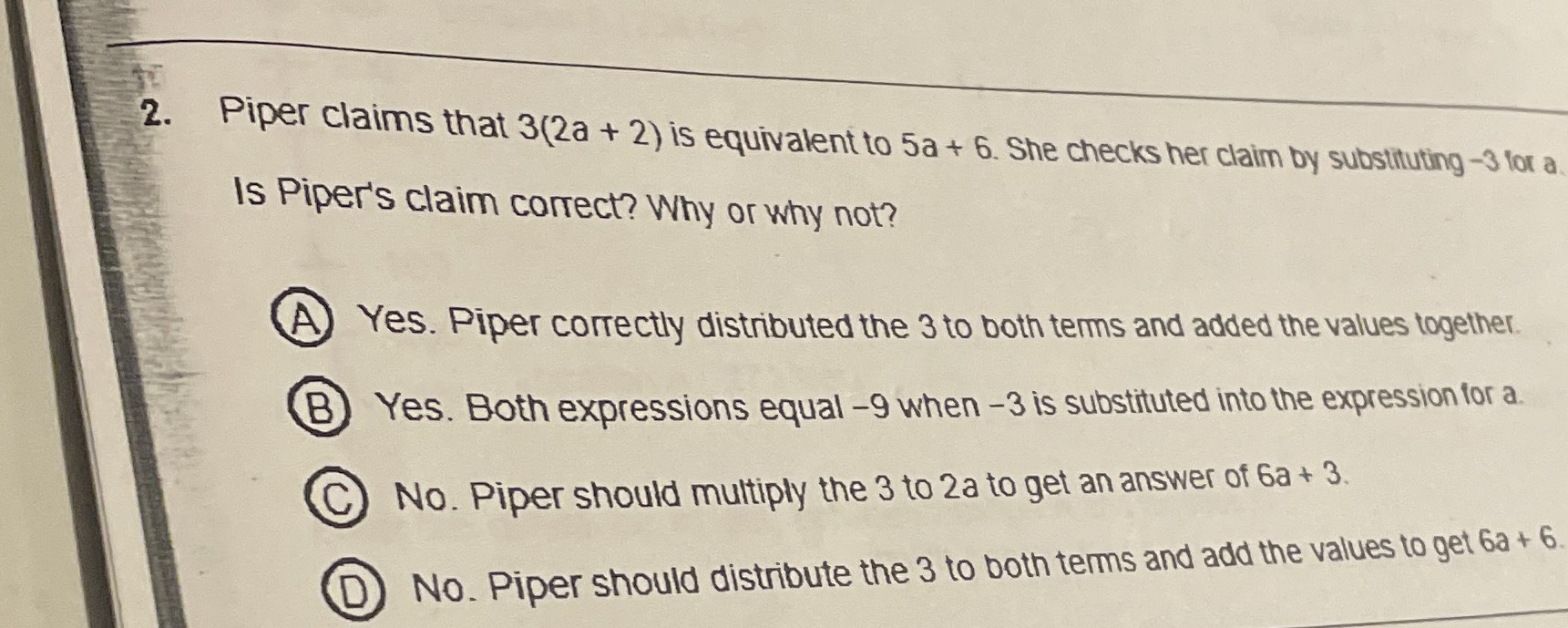### ¿Todavía tienes preguntas de matemáticas?

Pregunte a nuestros tutores expertos
Algebra
Pregunta2. Piper claims that $$3 ( 2 a + 2 )$$ is equivalent to $$5 a + 6$$ . She checks her claim by subsituting $$- 3$$ lor a. Is Piper's claim correct? Why or why not?

(A) Yes. Piper correctly distributed the $$3$$ to both terms and added the values together.

(B) Yes. Both expressions equal $$- 9$$ when $$- 3$$ is substituted into the expression for a.

C) No. Piper should multiply the $$3$$ to $$2 a$$ to get an answer of $$6 a + 3$$ .

(D) No. Piper should distribute the $$3$$ to both terms and add the values to get $$6 a + 6 .$$